Polynomials (Mathematics) Class 9 - NCERT Questions
Q 1.

Which of the following expressions are polynomials in one variable and which are not? State reasons for your answer.
(i) 4x² - 3x + 7 (ii)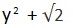(iii)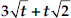(iv)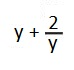(v)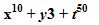SOLUTION:

(i) 4x² - 3x + 7 = 4x² - 3x + 7x0
4x² - 3x + 7 is a polynomial in one variable.
(ii)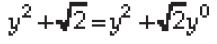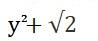is a polynomial in one variable.
(iii)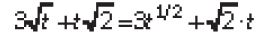Now,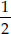is not a whole number.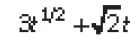is not a polynomial.
(iv)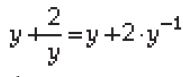Now, -1 is not a whole number.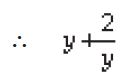is not a polynomial
(v) x10 + y³ + t50. Here, exponent of every variable is a whole number, but x10 + y³ + t50 is a polynomial in x, yand t, i.e. in three variables. So, it is not a polynomial in one variable.

Q 2.

Write the coefficients of x² in each of the following:
(i) 2 + x² + x (ii)2 - x² + x³
(iii)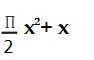(iv)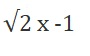SOLUTION:

(i) The given polynomial is 2 + x² + x. The coefficient of x² is 1.
4x² - 3x + 7 is a polynomial in one variable.
(ii)The given polynomial is 2 - x² +x³. The coefficient of x² is (-1). is a polynomial in one variable.
(iii)The given polynomial isThe coefficient of x² is p/2
(iv) The given polynomial isThe coefficient of x² is 0.

Q 3.

Give one example each of a binomial of degree 35, and of a monomial of degree 100.

SOLUTION:

(i) A binomial of degree 35 can be 3x35 - 4.
(ii) A monomial of degree 100 can be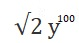The coefficient of x² is p/2

Q 4.

Write the degree of each of the following polynomials:
(i) 5x³ + 4x² + 7x (ii) 4 - y²
(iii)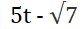(iv) 3

SOLUTION:

(i) The given polynomial is 5x³ + 4x² + 7x
The highest power of the variable x is 3. So, the degree of the polynomial is 3.
(ii) The given polynomial is 4 - y². The highest power of the variable y is 2.
So, the degree of the polynomial is 2.
(iii) The given polynomial isThe highest power of variable t is 1. So, the degree of the polynomial is 1.
(iv) Since, 3 = 3x0 [ x0 = 1]
So, the degree of the polynomial is 0.

Q 5.

Classify the following as linear, quadratic and cubic polynomials:
(i) x² + x (ii) x + x³
(iii) y + y² + 4 (iv) 1 + x
(v) 3t (vi) r²
(vii) 7x³

SOLUTION:

(i) The degree of x² + x is 2. So, it is a quadratic polynomial.
(ii) The degree of x - x³ is 3. So, it is a cubic polynomial.
(iii) The degree of y+ y² + 4 is 2. So, it is a quadratic polynomial.
(iv) The degree of 1 + x is 1. So, it is a linear polynomial.
(v) The degree of 3t is 1. So, it is a linear polynomial.
(vi) The degree of r² is 2. So, it is a quadratic polynomial.
(vii) The degree of 7x³ is 3. So, it is a cubic polynomial.

Q 6.

Find the value of the polynomial 5x - 4x² + 3 at
(i) x = 0 (ii) x = -1
(iii) x = 2

SOLUTION:

Let p(x) = 5x - 4x² + 3
(i) p(0) = 5(0) - 4(0)² + 3 = 0 - 0 + 3 = 3
Thus, the value of 5x - 4x² + 3 at x= 0 is 3.
(ii) p(-1) = 5(-1) - 4(-1)² + 3
= -5 - 4 + 3 = -9 + 3 = - 6
Thus, the value of 5x - 4x² + 3 at x= -1 is -6.
(iii) p(2) = 5(2) - 4(2)² + 3 = 10 - 4(4) + 3
= 10 - 16 + 3 = - 3
Thus, the value of 5x - 4x² + 3 at x= 2 is - 3.

Q 7.

Find p(0), p(1) and p(2) for each of the following polynomials:
(i) p(y) = y² - y + 1
(ii) p(t) = 2 + t + 2t² - t³
(iii) p(x) = x³
(iv) p(x) = (x - 1)(x + 1)

SOLUTION:

(i) Given that p(y) = y² - y+ 1.
p(0) = (0)² - (0) + 1 = 0 - 0 + 1 = 1,
p(1) = (1)² - (1) + 1 = 1 - 1 + 1 = 1,
p(2) = (2)² - 2 + 1 = 4 - 2 + 1 = 3
(ii) Given that p(t) = 2 + t + 2t² - t³
p(0) = 2 + (0) + 2(0)² - (0)3
= 2 + 0 + 0 - 0 = 2,
p(1) = 2 + (1) + 2(1)² - (1)3
= 2 + 1 + 2 - 1 = 4,
p(2) = 2 + 2 + 2(2)² - (2)3
= 2 + 2 + 8 - 8 = 4
(iii) Given that p(x) = x³
p(0) = (0)³ = 0, p(1) = (1)³ = 1,
p(2) = (2)³ = 8
(iv) Given that p(x) = (x- 1)(x + 1)
p(0) = (0 - 1)(0 + 1) = -1 x 1 = -1,
p(1) = (1 - 1)(1 + 1) = (0)(2) = 0,
p(2) = (2 - 1)(2 + 1) = (1)(3) = 3

Q 8.

Verify whether the following are zeroes of the polynomial, indicated against them.
(i)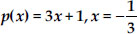(ii)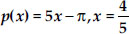(iii) p(x) = x² - 1, x = 1, -1
(iv) p(x) = (x + 1)(x - 2), x = -1, 2
(v) p(x) = x², x = 0
(vi)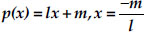(vii)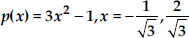(viii)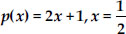SOLUTION:

(i) Given that p(x) = 3x + 1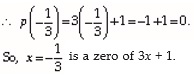(ii) Given that p(x) = 5x - p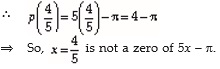(iii) Given that p(x) = x² - 1,
p(1) = (1)² - 1 = 1 - 1 = 0
Since, p(1) = 0, So x= 1 is a zero of x² - 1.
Also, p(-1) = (-1)² - 1 = 1 - 1 = 0
Since p(-1) = 0
x= -1 is also a zero of x² - 1.
(iv) We have, p(x) = (x + 1)(x - 2)
p(-1) = (-1 + 1) (- 1 - 2) = (0)(- 3) = 0
Since, p(-1) = 0
⇒ x= -1 is a zero of (x + 1)(x- 2).
Also, p(2) = (2 + 1)(2 - 2) = (3)(0) = 0
Since, p(2) = 0
⇒ x= 2 is also a zero of (x + 1)(x- 2).
(v) We have, p(x) = x² ⇒ p(0) = (0)² = 0. Since, p(0) = 0; x = 0 is a zero of x².
(vi) We have, p(x) = lx+ m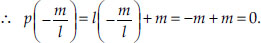Since,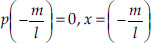is a zero of lx + m.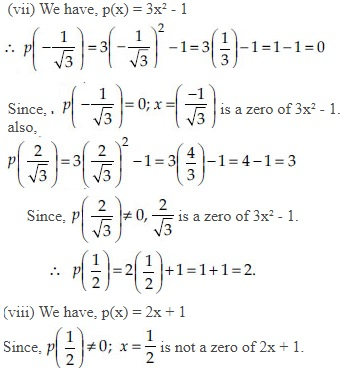Q 9.

Find the zero of the polynomial in each of the following cases:
(i) p (x ) = x + 5 (ii) p (x) = x - 5
(iii) p (x) = 2x + 5 (iv) p (x) = 3x - 2
(v) p (x) = 3x (vi) p (x) = ax , a ≠ 0
(vii) p (x) = cx + d , c ≠ 0, c, d are real numbers.

SOLUTION:

(i) We have, p(x) = x+ 5. Since, p(x) = 0
⇒ x + 5 = 0 ⇒ x = - 5.
Thus, zero of x+ 5 is -5.
(ii) We have, p(x) = x- 5. Since, p(x) = 0
⇒ x - 5 = 0 ⇒ x = 5
Thus, zero of x- 5 is 5.
(iii) We have, p(x) = 2x + 5. Since, p(x) = 0
⇒ 2x + 5 = 0 ⇒ 2x = -5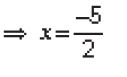Thus, zero of 2x + 5 is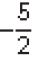(iv) We have, p(x) = 3x - 2.
Since, p(x) = 0 ⇒ 3x = 2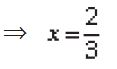Thus, zero of 3x - 2 is 2.
Since, p(x) = 0 ⇒ 3x = 2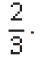(v) We have, p(x) = 3x.
Since, p(x) = 0 ⇒ 3x = 0 ⇒ x = 0
Thus, zero of 3x is 0.
(vi) We have, p(x) = ax,a ≠ 0.
Since, p(x) = 0 ⇒ ax = 0 ⇒ x= 0
Thus, zero of axis 0.
(vii) We have, p(x) = cx + d. Since, p(x) = 0
⇒ cx + d = 0 ⇒ cx = - d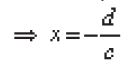Thus, zero of cx + d is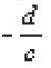Q 10.

Find the remainder when x³ + 3x² + 3x + 1 is divided by
(i) x + 1 (ii)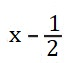(iii) x (iv) x + π
(v) 5 + 2x

SOLUTION:

Let p(x) = x³ + 3x² + 3x + 1
(i) The zero of (x + 1) is - 1. So, by remainder theorem, p(-1) is the remainder when p(x) is divided by x+ 1.
p(-1) = (-1)3 + 3(-1)² + 3(-1) + 1
= -1 + (3 x 1) + (-3) + 1
= -1 + 3 - 3 + 1 = 0
Thus, the required remainder = 0
(ii) The zero of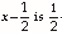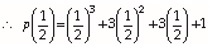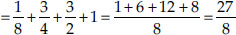Thus, the required remainder =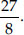(iii) The zero of x is 0.
p(0) = (0)3 + 3(0)² + 3(0) + 1
= 0 + 0 + 0 + 1 = 1
Thus, the required remainder = 1.
(iv) The zero of x+ p is (-p).
p(-p) = (-p)³ + 3(- p)² + 3(- p) + 1
= - p³+ 3p² + (-3p) + 1
= - p³ + 3p² - 3p + 1
Thus, the required remainder is
-p³ + 3p² - 3p + 1.
(v) The zero of 5 + 2x is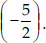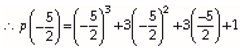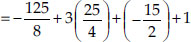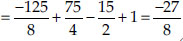Thus, the required remainder is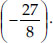Q 11.

Find the remainder when x³ - ax² + 6x - a is divided by x - a.

SOLUTION:

We have, p(x) = x³ - ax² + 6x - a and zero of x- ais a.
p(a) = (a)³ -a(a)² + 6(a) - a
= a³ - a³ + 6a -a = 5a
Thus, the required remainder = 5a

Q 12.

Check whether 7 + 3x is a factor of 3x³ + 7x.

SOLUTION:

We have, p(x) = 3x³ + 7x and zero of 7 + 3x is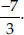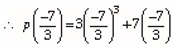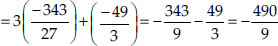Since,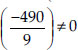i.e. the remainder is not 0.
3x³ + 7x is not divisible by 7 + 3x.
Thus, (7 + 3x) is not a factor of 3x³ + 7x.

Q 13.

Determine which of the following polynomials has (x + 1) a factor :
(i) x³ + x² + x + 1
(ii) x4 + x³ + x² + x + 1
(iii) x4 + 3x³ + 3x² + x + 1
(iv) x3 - x2 - (2 + √2) x + √2

SOLUTION:

The zero of x+ 1 is -1.
(i) Let p(x) = x³ + x³ + x+ 1
p(-1) = (-1)³ + (-1)³ + (-1) + 1
= -1 + 1 - 1 + 1 = 0
⇒ p(-1) = 0, so (x+ 1) is a factor of x³ + x³ + x+ 1.
(ii) Let p(x) = x4 + x³ +x² + x+ 1
p(-1) = (-1)4 + (-1)³ + (-1)²+ (-1) + 1
= 1 - 1 + 1 - 1 + 1 = 1
⇒ p(-1) ≠ 0, so (x + 1) is not a factor of x4 + x³+ x² + x+ 1.
(iii) Let p(x) = x4 + 3x³ + 3x² + x+ 1
p(-1) = (-1)4 + 3(-1)³ + 3(-1)² + (-1) + 1
= 1 - 3 + 3 - 1 + 1 = 1
⇒ p(-1) ≠ 0, so (x + 1) is not a factor of
x4 + 3x³ + 3x² + x+ 1.
(iv)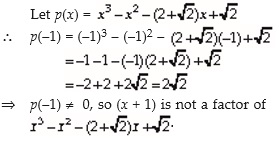Q 14.

Use the Factor theorem, to determine whether g(x) is a factor of p(x) in each of the following cases:
(i) p (x) = 2x³ + x² - 2x - 1, g (x) = x + 1
(ii) p (x) = x³ + 3x² + 3x + 1, g (x) = x + 2
(iii) p (x) = x³ - 4x² + x + 6, g (x) = x - 3

SOLUTION:

(i) We have, p(x) = 2x³ + x² - 2x - 1 and
g(x) = x+ 1
p(-1) = 2(-1)³ + (-1)² - 2(-1) - 1
= 2(-1) + 1 + 2 - 1 = - 2 + 1 + 2 - 1 = 0
⇒ p(-1) = 0, so g(x) is a factor of p(x).
(ii) We have, p(x) = x³ + 3x² + 3x + 1 and g(x) = x+ 2
p(-2) = (-2)³ + 3(-2)² + 3(-2) + 1
= -8 + 12 - 6 + 1 = -14 + 13 = - 1
⇒ p(-2) ≠ 0, so g(x) is not a factor of p(x).
(iii) We have, p(x) = x³ - 4x² + x+ 6 and g(x) = x - 3
p(3) = (3)³ - 4(3)² + 3 + 6
= 27 - 4(9) + 3 + 6
= 27 - 36 + 3 + 6 = 0
⇒ p(3) = 0, so g(x) is a factor of p(x).

Q 15.

Find the value of k, if x - 1 is a factor of p(x) in each of the following cases :
(i) p (x) = x² + x + k
(ii)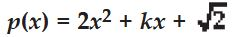(iii)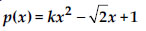(iv) p (x) = kx² - 3x + k

SOLUTION:

For (x- 1) be a factor of p(x), p(1) should be equal to 0. (i) Here, p(x) = x² + x + k Since, p(1) = (1)² + 1 + k⇒ p(1) = k+ 2 = 0 ⇒ k + 2 = 0 ⇒ k = -2. (ii) Here, p(x) = 2x² + kx+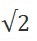Since, p(1) = 2(1)² + k(1) += 2 + k += 0
⇒k + 2 += 0 ⇒k = -2-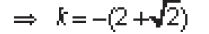(iii) Here, p(x) = kx² -x + 1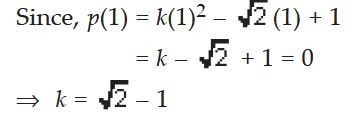(iv) Here, p(x) = kx2 - 3x + k
p(1) = k(1)² - 3(1) + k=k - 3 + k
= 2k - 3 = 0
⇒ 2k - 3 = 0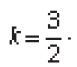Q 16.

Factorise :
(i) 12x² - 7x + 1 (ii) 2x² + 7x + 3
(iii) 6x² + 5x - 6 (iv) 3x² - x - 4

SOLUTION:

(i) We have,
12x² - 7x + 1 = 12x² - 4x - 3x + 1
= 4x(3x - 1) - 1(3x - 1) = (3x - 1)(4x - 1)
Thus, 12x² - 7x + 1 = (3x - 1)(4x - 1)
(ii) We have, 2x² + 7x + 3 = 2x² + x+ 6x + 3
=x(2x + 1) + 3(2x + 1) = (2x + 1)(x + 3)
Thus, 2x² + 7x+ 3 = (2x + 1)(x + 3)
(iii) We have, 6x² + 5x - 6 = 6x² + 9x - 4x - 6
= 3x(2x + 3) - 2(2x + 3) = (2x + 3)(3x - 2)
Thus, 6x² + 5x - 6 = (2x + 3)(3x - 2)
(iv) We have, 3x² - x- 4 = 3x² - 4x + 3x - 4
= x(3x - 4) + 1(3x - 4) = (3x - 4)(x + 1)
Thus, 3x² - x- 4 = (3x - 4)(x + 1)

Q 17.

Factorise :
(i) x³ - 2x² - x + 2
(ii) x³ - 3x² - 9x - 5
(iii) x³ + 13x² + 32x + 20
(iv) 2y³ + y² - 2y - 1

SOLUTION:

(i) We have, x³ - 2x² - x+ 2
Rearranging the terms, we have
x³ - 2x² - x + 2 = x³ - x- 2x² + 2
= x(x² - 1) - 2(x² - 1) = (x² - 1)(x - 2)
= [(x)² - (1)²](x - 2)
[ (a² - b²) = (a+ b)(a - b)]
= (x- 1)(x + 1)(x - 2)
Thus, x³ - 2x² - x + 2 = (x - 1)(x + 1)(x - 2)
(ii) We have, x³ - 3x² - 9x - 5
= x³ +x² - 4x² - 4x - 5x - 5
= x²(x + 1) - 4x(x + 1) - 5(x + 1)
= (x + 1)(x² - 4x - 5)
= (x + 1)(x² - 5x + x- 5)
= (x + 1)[x(x - 5) + 1(x - 5)]
= (x + 1)(x - 5)(x + 1)
Thus, x³ - 3x² - 9x - 5 = (x + 1)(x - 5)(x + 1)
(iii) We have, x³ + 13x² + 32x + 20
= x³ + x² + 12x² + 12x + 20x + 20
= x²(x + 1) + 12x(x + 1) + 20(x + 1)
= (x + 1)(x² + 12x + 20)
= (x + 1)(x² + 2x + 10x + 20)
= (x + 1)[x(x + 2) + 10(x + 2)]
= (x + 1)(x + 2)(x + 10)
Thus, x³ + 13x² + 32x + 20
= (x + 1)(x + 2)(x + 10)
(iv) We have, 2y³ + y² - 2y - 1
= 2y³ - 2y² + 3y² - 3y + y - 1
= 2y²(y - 1) + 3y(y - 1) + 1(y - 1)
= (y - 1)(2y² + 3y + 1)
= (y - 1)(2y² + 2y + y+ 1)
= (y - 1)[2y(y + 1) + 1(y + 1)]
= (y - 1)(y + 1)(2y + 1)
Thus, 2y³ + y² - 2y - 1 = (y - 1)(y + 1)(2y + 1)

Q 18.

Use suitable identities to find the following products:
(i) (x + 4)(x + 10)
(ii) (x + 8)(x - 10)
(iii) (3x + 4)(3x - 5)
(iv)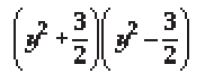(v) (3 - 2x )(3 + 2x )

SOLUTION:

(i) We have, (x+ 4)(x + 10)
Using the identity
(x + a)(x +b) = x² + (a +b)x + ab, we have
(x + 4)(x + 10) = x² + (4 + 10)x + (4 × 10)
= x² + 14x + 40
(ii) We have, (x + 8)(x – 10). Here, a= 8 and b= –10
Using the identity,
(x+ a)(x+ b) = x² + (a + b)x + ab, we have
(x + 8)(x – 10) = x² + [8 + (–10)]x + [8 × (–10)] = x² + (–2)x + (–80) = x² – 2x – 80
(iii) We have, (3x + 4)(3x – 5)
Using the identity,
(x+ a)(x+ b) =x² + (a + b)x + ab, we have
(3x + 4)(3x – 5)
= (3x)² + [4 + (–5)]3x + [4 × (–5)]
= 9x² + (–1)3x + (–20) = 9x² – 3x –20
(iv) We have,Using the identity,
(a + b)(a –b) = a² – b², we have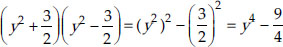(v) We have, (3 – 2x)(3 + 2x)
Using the identity, (a+ b)(a – b) = a² – b², we have
(3 – 2x)(3 + 2x) = (3)² – (2x)² = 9 – 4x²

Q 19.

Evaluate the following products without multiplying directly :
(i) 103 x 107
(ii) 95 x 96
(iii) 104 x 96

SOLUTION:

(i) We have, 103 x 107 = (100 + 3)(100 + 7)
= (100)² + (3 + 7) x 100 + (3 x 7) [Using (x+ a)(x+ b) = x² + (a + b)x + ab]
= 10000 + (10) x 100 + 21
= 10000 + 1000 + 21= 11021
(ii) We have, 95 x 96 = (100 - 5)(100 - 4)
= (100)² + [(-5) + (- 4)] x 100 + [(-5) x (-4)] [Using (x+ a)(x + b) = x² + (a + b)x +ab]
= 10000 + (-9) x 100 + 20
= 10000 + (-900) + 20 = 9120
(iii) We have, 104 x 96 = (100 + 4)(100 - 4)
= (100)² - (4)²
[Using (a+ b)(a - b) =a² - b²]
= 10000 - 16 = 9984

Q 20.

Factorise the following using appropriate identities :
(i) 9x² + 6xy + y²
(ii) 4y² - 4y + 1
(iii)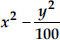SOLUTION:

i) We have, 9x² + 6xy + y² = (3x)² + 2(3x)(y) + (y)² = (3x + y)² = (3x + y)(3x + y) [Using a² + 2ab + b² = (a + b)²] (ii) We have, 4y² - 4y + 1 = (2y)v - 2(2y)(1) + (1)² = (2y - 1)² = (2y - 1)(2y - 1) [Using a² - 2ab + bv = (a - b)²] (iii) We have,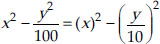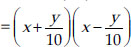[Using a² - b² = (a+ b)(a - b) ]

Q 21.

Expand each of the following, using suitable identities :
(i) (x + 2y + 4z)²
(ii) (2x - y + z )²
(iii) (-2x + 3y + 2z )²
(iv) (3a - 7b - c )²
(v) (-2x + 5y - 3z )²
(vi)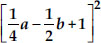SOLUTION:

We know that
(x + y + z)² =x² + y² + z² + 2xy + 2yz + 2zx
(i) (x + 2y + 4z)² = (x)² + (2y)² + (4z)²
+ 2(x)(2y) + 2(2y)(4z) + 2(4z)(x)
= x² + 4y² + 16z² + 4xy + 16yz + 8zx
(ii) (2x - y+ z)² = (2x)² + (-y)² + (z)²
+ 2(2x)(-y) + 2(-y)(z) + 2(z)(2x)
= 4x² + y² + z² - 4xy - 2yz + 4zx
(iii) (-2x+ 3y + 2z)² = (-2x)² + (3y)² + (2z)²
+ 2(-2x)(3y) + 2(3y)(2z) + 2(2z)(-2x)
= 4x² + 9y² + 4z² - 12xy + 12yz - 8zx
(iv) (3a - 7b -c)² = (3a)² + (-7b)² + (-c)²
+ 2(3a)(-7b) + 2(-7b)(-c) + 2(-c)(3a)
= 9a² + 49b² +c² - 42ab + 14bc - 6ca
(v) (-2x + 5y - 3z)² = (-2x)² + (5y)² + (-3z)²
+ 2(-2x)(5y) + 2(5y)(-3z) + 2(-3z)(-2x)
= 4x² + 25y² + 9z² - 20xy -30yz + 12zx
(vi)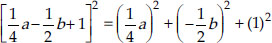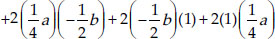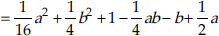Q 22.

Factorise:
(i) 4x² + 9y² + 16z² + 12xy - 24yz - 16xz
(ii)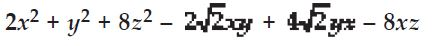SOLUTION:

(i) We have,
4x² + 9y² + 16z² + 12xy - 24yz- 16xz
= (2x)² + (3y)² + (-4z)² + 2(2x)(3y)
+ 2(3y)(-4z) + 2(-4z)(2x)
= (2x + 3y - 4z)² = (2x + 3y - 4z)(2x + 3y - 4z)
(ii)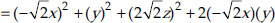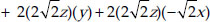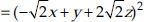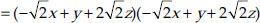Q 23.

Write the following cubes in expanded form: (i) (2x + 1)³ (ii) (2a - 3b )³
(iii)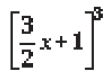(iv)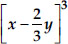SOLUTION:

(We have (x + y)³ = x³ + y³ + 3xy(x + y) ... (1)
and (x - y)³ = x³ - y³ - 3xy(x - y) ... (2)
(i) (2x + 1)³ = (2x)³ + (1)³ +
3(2x)(1)(2x + 1) [By (1)]
= 8x³ + 1 + 6x(2x + 1) = 8x³ + 12x² + 6x + 1
(ii) (2a - 3b³ = (2a)³ - (3b)³ - 3(2a)(3b)(2a - 3b)
[By (2)]
= 8a³ - 27b³ - 18ab(2a - 3b)
= 8a³ - 27b³ - (36a²b - 54ab²)
= 8a³ - 27b³ - 36a²b + 54ab²
(iii)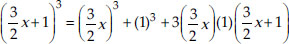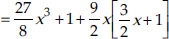[By(1)]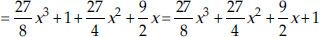(iv)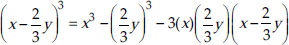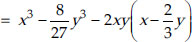[By (2)]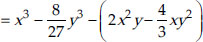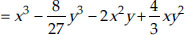Q 24.

Evaluate the following using suitable identities:
(i) (99)³ (ii) (102)³
(iii) (998)³

SOLUTION:

(i) We have, 99 = (100 - 1)
99³ = (100 - 1)³
= (100)³ - 13 - 3(100)(1)(100 - 1)
= 1000000 - 1 - 300(100 - 1)
= 1000000 - 1 - 30000 + 300 = 1000300 - 30001 = 970299
(ii) We have, 102 = 100 + 2
102³ = (100 + 2)³
= (100)³ + (2)³ + 3(100)(2)(100 + 2)
= 1000000 + 8 + 600(100 + 2)
= 1000000 + 8 + 60000 + 1200 = 1061208
(iii) We have, 998 = 1000 - 2
(998)³ = (1000 - 2)³
= (1000)³ - (2)³ - 3(1000)(2)(1000 - 2)
= 1000000000 - 8 - 6000(1000 - 2)
= 1000000000 - 8 - 6000000 + 12000
= 994011992

Q 25.

Factorise each of the following :
(i) 8a³ + b³ + 12a²b + 6ab²
(ii) 8a³ - b³ - 12a²b + 6ab*2
(iii) 27 - 125a*3 - 135a + 225a²
(iv) 64a³ - 27b³ - 144a²b + 108ab²
(v)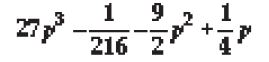SOLUTION:

(i) 8a³ + b³ + 12a²b + 6ab²
= (2a)³ + (b)³ + 6ab(2a +b)
= (2a)³ + (b)³ + 3(2a)(b)(2a + b)
= (2a +b)³ = (2a + b)(2a + b)(2a+ b)
(ii) 8a³ - b³ - 12a²b + 6ab²
= (2a)³ - (b)³ - 3(2a)(b)(2a- b)
= (2a- b)³ = (2a -b)(2a - b)(2a -b)
(iii) 27 - 125a³ - 135a + 225a²
= (3)3 - (5a)³ - 3(3)(5a)(3 - 5a)
= (3 - 5a)³ = (3 - 5a)(3 - 5a)(3 - 5a)
(iv) 64a³ - 27b³ - 144a²b + 108ab²
= (4a)³ - (3b)³ - 3(4a)(3b)(4a - 3b)
= (4a - 3b)³ = (4a - 3b)(4a - 3b)(4a - 3b)
(v)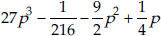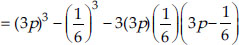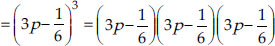Q 26.

Verify : (i) x³ + y³ = (x + y)(x² - xy + y²)
(ii) x³ - y³ = (x - y)(x² + xy + y²)

SOLUTION:

(i) (x + y)³ = x³ + y³ + 3xy(x + y)
⇒ (x +y)³ - 3(x+ y)(xy) = x³ + y³
⇒ (x + y)(x² + y² - xy) = x³ + y³
Hence verified.
(ii) (x -y)³ =x³ - y³ - 3xy(x - y)
⇒ (x - y)³ + 3xy(x -y) = x³ - y³
⇒ (x - y)³ + 3xy(x - y) = x³ - y³
⇒ (x -y)((x- y)² + 3xy) = x³ - y³
⇒ (x -y)(x² + y² + xy) = x³ - y³
Hence, verified.

Q 27.

Factorise each of the following :
(i) 27y³ + 125z³ (ii) 64m³ - 343n³

SOLUTION:

(i) We know that
(x³ + y³) = (x+ y)(x² - xy+ y²)
We have, 27y³ + 125z³ = (3y)³ + (5z)³
= (3y + 5z)[(3y)² - (3y)(5z) + (5z)²]
= (3y + 5z)(9y² - 15yz + 25z2)
(ii) We know that
x³ - y³ = (x - y)(x² + xy+ y²)
We have, 64m³ - 343n³ = (4m)³ - (7n)³
= (4m - 7n)[(4m)² + (4m)(7n) + (7n)²]
= (4m - 7n)(16m² + 28mn + 49n²)

Q 28.

Factorise : 27x³ + y³ + z³ - 9xyz

SOLUTION:

We have, 27x³ +y³ +z³ - 9xyz
= (3x)³ + (y)³ + (z)³ - 3(3x)(y)(z)
Using the identity,
x³ + y³ + z³ - 3xyz = (x +y + z)(x² + y² + z²
- xy- yz- zx)
We have, (3x)³ + (y)³ + (z)³ - 3(3x)(y)(z)
= (3x + y +z)[(3x)² +y² +z² - (3x x y)
- (y x z) - (z x 3x)]
= (3x + y+ z)(9x² + y² + z² - 3xy - yz - 3zx)

Q 29.

Verify that x³ + y³ + z³ - 3xyz = (x + y + z) [(x - y)² + (y - z)² + (z - x)²]

SOLUTION:

R.H.S.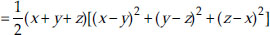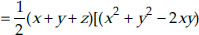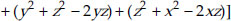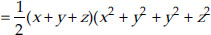+ z2 + x² - 2xy - 2yz - 2zx)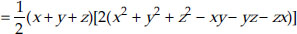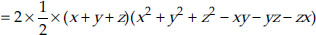= (x + y + z)(x² + y² + z² - xy- yz-zx) = x³ + y³ + z³ - 3xyz = L.H.S. Hence, verified.

Q 30.

If x + y + z = 0, then show that
x³ + y³ + z³ = 3xyz

SOLUTION:

Since, x+ y+ z= 0
⇒ x + y = - z ⇒ (x +y)³ = (-z)3
⇒ x³ + y³ + 3xy(x + y) = -z³
⇒ x³ + y³ + 3xy(-z) = -z³ [ x + y = (-z)]
⇒ x³ + y³ - 3xyz = -z³
⇒ x³ + y³ + z³ = 3xyz
Hence, if x + y + z = 0, then
x³ + y³ + z³ = 3xyz

Q 31.

Without actually calculating the cubes, find the value of each of the following :
(i) (-12)³ + (7)³ + (5)³
(ii) (28)³ + (-15)³ + (-13)³

SOLUTION:

(i) We have, (-12)³ + (7)³ + (5)³
Let x = -12, y= 7 and z= 5.
Then, x + y + z = -12 + 7 + 5 = 0
We know that if x + y + z = 0,
then, x³ + y³ + z³ = 3xyz
(-12)³ + (7)³ + (5)³ = 3[(-12)(7)(5)]
= 3[-420] = -1260
(ii) (28)³ + (-15)³ + (-13)³
Let x= 28, y= -15 and z= -13. Then,
x + y + z = 28 - 15 - 13 = 0
We know that if x+ y+ z= 0, then
x³ + y³ + z³ = 3xyz
(28)³ + (-15)³ + (-13)³ = 3(28)(-15)(-13)
= 3(5460) = 16380

Q 32.

Give possible expressions for the length and breadth of each of the following rectangles, in which their areas are given :
(i)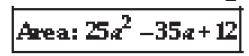(ii)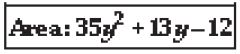SOLUTION:

Area of a rectangle = (Length) x (Breadth)
(i) 25a² - 35a + 12 = 25a² - 20a - 15a + 12
= 5a(5a - 4) - 3(5a - 4) = (5a - 4)(5a - 3)
Thus, the possible length and breadth are (5a - 3) and (5a - 4).
(ii) 35y² + 13y - 12 = 35y² + 28y - 15y - 12
= 7y(5y + 4) - 3(5y + 4) = (5y + 4)(7y - 3)
Thus, the possible length and breadth are
(7y - 3) and (5y + 4).

Q 33.

What are the possible expressions for the dimensions of the cuboids whose volumes are given by?
(i)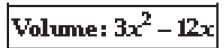(ii)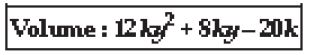SOLUTION:

Volume of a cuboid = (Length) x (Breadth)
x (Height)
(i) Volume = 3x² - 12x
We have 3x² - 12x = 3(x² - 4x)
= 3 x x x (x - 4)
The possible dimensions of the cuboid are 3, x and (x - 4).
(ii) Volume = 12ky² + 8ky - 20k
We have, 12ky² + 8ky - 20k
= 4[3ky² + 2ky - 5k] = 4[k(3y² + 2y - 5)]
= 4 x k x (3y² + 2y - 5)
= 4k[3y² - 3y + 5y - 5]
= 4k[3y(y - 1) + 5(y - 1)]
= 4k[(3y + 5) x (y - 1)]
= 4k x (3y + 5) x (y - 1)
Thus, the possible dimensions of the cuboid
are 4k, (3y + 5) and (y- 1).